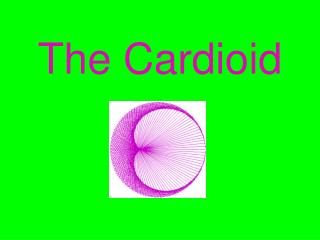Download PresentationThe Cardioid

# The Cardioid - PowerPoint PPT PresentationDownload Presentation## The Cardioid

- - - - - - - - - - - - - - - - - - - - - - - - - - - E N D - - - - - - - - - - - - - - - - - - - - - - - - - - -
##### Presentation Transcript

1. The Cardioid

2. DESCRIPTION: • The word ‘cardioid’ comes from the Greek root ‘cardi’ meaning heart. The Cardioid curve is a special case of the epicycloid and the limacon of Pascal. It can also be defined as the curve traced by a point of a circle that rolls around the circumference of a fixed circle of equal radius without slipping.

3. History • Studied by Ole Christensen Roemer in 1674, it was discovered during an effort to try to find the best design for gear teeth. The curve was given its name by de Castillon in the philosophical ransaction of the Royal Society of 1741. The arc length was later discovered in 1708 by La Hire. • However, since this cardioid is also a special case of the limacon of Pacscal, it is believed by some to have been originated from Etiene Pascal’s studies. (1588-1640)

4. Start with two circles with centers C¹ and C² with radius R. • Circle C² is tangent to circle C¹ at point T and center C² is 2R from C¹.

5. Let angle TC¹D be the angle made by the line going through the centers of the two circles and the x-axis. Let it be known as q1in our explanation. • So far, we know that the coordinates of C² are as follows:

6. To find the coordinates of the point A we can do what we did previously, but we have two unknown angles AC²E(q3) and AC²B(q²). • Draw a line parallel to the x-axis through C² and point D (L1).

7. Now draw two adjacent isosceles-right triangles with their height being the distance from point T to the x-axis at point H, their bases are each R (along the segment C¹ to C²), and their hypotenuse be the distance from each circle’s center to point H.

8. Using the SAS theorem (side-angle-side) we know that since these two triangles share the right angle, their base and their height, that their other angles must be equal. • In turn, using another geometric proof, we now can see that q³ is equal to the sum of q¹ and q², AND that q¹ and q² are equal.

9. Finally, let t be equal to the angle q¹. • Therefore, the parametric equations (and coordinates of the point A that travels around the circle) for the Cardioid are:

10. Cardioid Using Parametric Equations • t=linspace(0,2*pi) • R=1; • x=2*R*cos(t)+R*cos(2*t) • y=2*R*sin(t)+R*sin(2*t) • plot(x,y) • grid on • axis square • title('The Cardioid Using Parametric Equations')

11. Cardioid Using Polar Coordinates • theta=linspace(0,2*pi); • r=2*(1+cos(theta)); • polar(theta,r) • title('The Cardioid Using Polar Coordinates')

12. LOCUS!

13. Sources: • Xah: Special Place Curveshttp://www.best.com/~xah/SpecialPlaneCurves_dir/Cardioid_dir/cardioid.html • Peter Gent: Cardioidhttp://online.redwoods.cc.ca.us/instruct/darnold/CalcProj/Sp98/PeterG/Cardioid.html • Sean Larson: Introducing the Cardioidhttp://online.redwoods.cc.ca.us/instruct/darnold/CalcProj/Sp98/seanL/cardioidf.html • Limaconhttp://mathworld.wolfram.com/Limacon.html • Cardioidhttp://en.wikipedia.org/wiki/Cardioid